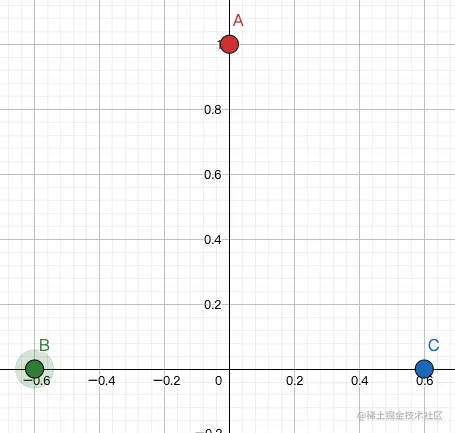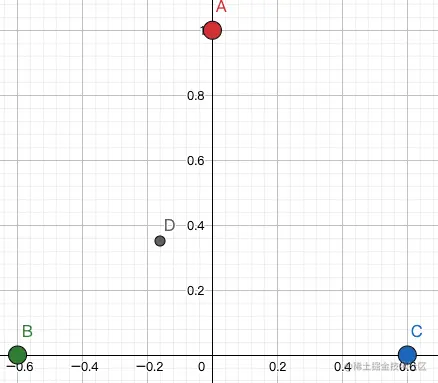# Webgl lesson 25: basic knowledge of mapping August challenge

2021-08-27 08:42:02

This is my participation 8 The fourth of the yuegengwen challenge 11 God , Check out the activity details ：8 Yuegengwen challenge

 In this paper, the title ：WebGL Lesson 25 ： The basics of mapping ｜ 8 Yuegengwen challenge
Copy code 

### Friendship tips

This article is WebGL Course column Of the 25 piece , It is strongly recommended to start from the front . Because I spent a lot of time explaining the concept of vector and matrix operation . These basics will affect your thinking .

### Introduction

We know a picture , Even if it's small , There are thousands of pixels .

But we are WebGL The number of vertices , Usually not so much .

such as , Use the way we did last time , Draw a square , It was introduced 12 A little bit  nothing more .( If you think it's a four point , You can go back to the last class and take a look at , Then taste the content of this class )

that , If we need to fill this square with a picture , What to do .

We can never , A pixel , Just prepare a vertex , It won't explode yet .

So , Still want to review WebGL The principle of drawing triangles ：

1. Position the three points of the triangle
1. According to the incoming color , Or according to shader The color in , Draw the dots
1.  interpolation ( The point is this sentence )

### Interpolation in-depth explanation

#### Two point interpolation

What is two-point interpolation ？ in other words , Now there are two points : $A (x_A, y_A)$ and $B(x_B, y_B)$.

spot A The color of the is RGB To express is to (1,1,1), That is, pure white .

spot B The color of the is RGB To express is to (0,0,0), That is, pure black .

 The problem is coming. , take AB Connect , ask AB What is the color of any point on the line segment ?
Copy code 

We use our  A simple feeling  To come to a conclusion ：

 He who is near to the red, he who is near to the black ,  That is to say   near A White   near B Black
Copy code 

The specific formula will not be listed , When you need it , Then list .

#### Three point interpolation

This will take a little time to say . Suppose there are three points : ABC, The colors are : red green blue .

Like the picture below ：Okay , So these three points , Inside the triangle , Any point , For example, it , Let's make a point D come out , So this point D, If you use interpolation , What color should it be ?Let's give an interesting conclusion ：

If ABC The coordinates of the three points are

$\left(\begin{array}{cc} x_A\\ y_A\end{array}\right) \left(\begin{array}{cc} x_B\\ y_B\end{array}\right) \left(\begin{array}{cc} x_C\\ y_C\end{array}\right)$

If D The coordinates of point

$\left(\begin{array}{cc} x_D\\ y_D\end{array}\right) = a * \left(\begin{array}{cc} x_A\\ y_A\end{array}\right) + b * \left(\begin{array}{cc} x_B\\ y_B\end{array}\right)+ c * \left(\begin{array}{cc} x_C\\ y_C\end{array}\right)$ ( type 1)

that D The color of the point

$D_ Yan = a * A_ Yan + b * B_ Yan + c * C_ Yan$ ( type 2)

in other words ： We find Three numbers a b c. If these three numbers can ABC The coordinates of are combined into D The coordinates of point , that D The color of the point , That is, these three numbers will ABC Combine the colors of .

Use a matrix to express  type 1, That is to say D The point coordinates are obtained ：

$\left(\begin{array}{cc} x_D\\ y_D\end{array}\right) = \left(\begin{array}{ccc} x_A&x_B&x_C\\ y_A&y_B&y_C\end{array}\right) * \left(\begin{array}{cc} a\\ b \\c \end{array}\right)$

Use a matrix to express  type 2, That is to say D Point color to get ：

$\left(\begin{array}{cc} R_D\\ G_D\\ B_D\end{array}\right) = \left(\begin{array}{ccc} R_A&R_B&R_C\\ G_A&G_B&G_C\\ B_A&B_B&B_C\end{array}\right) * \left(\begin{array}{cc} a\\ b \\c \end{array}\right)$

Formally speaking , But everything ABC What three points have , Whatever you are , Or color , Or something else , All can be used abc These three numbers are combined .

1. For example ,  temperature

ABC The temperatures at the three points are 1℃, 30℃, 50 ℃. It is known that D Point coordinates ：

$\left(\begin{array}{cc} x_D\\ y_D\end{array}\right) = \left(\begin{array}{ccc} x_A&x_B&x_C\\ y_A&y_B&y_C\end{array}\right) * \left(\begin{array}{cc} a\\ b \\c \end{array}\right)$

ask ： If the interpolation algorithm is used , that D What should be the temperature at the point ？

answer ： We use it abc Combine three numbers .

$\left(\begin{array}{cc} temperature _D\end{array}\right) = \left(\begin{array}{ccc} temperature _A& temperature _B& temperature _C\end{array}\right) * \left(\begin{array}{cc} a\\ b \\c \end{array}\right) = a * 1 + b * 30 + c * 50$

### Sampling information ：UV

Need to know , The purpose of our class , It's not a simple synthesis of a color .

Instead, fill a picture in the middle .

It is necessary to add a step , It's our introduction WebGL The point of , How does it correspond to the picture itself .

We know a picture , It's just one. width * height The rectangular area of .

Let's put this rectangular area

• The lower left corner is regarded as the origin (0,0)

• In the upper right corner (1,1) Words

So anywhere in the middle , All have a coordinate , Let's write this coordinate as (u, v).

for example ,

• In the center of the picture (u, v) = (0.5, 0.5)
• Upper left corner (u, v) = (0, 1)
• Right lower corner (u, v) = (1, 0)

in other words , Any one (u,v) Can be on a picture , Find a place , And this location must have the image itself RGB Color information .

This is how we specify the picture information of a coordinate point , The message is UV Information .

for example ,A Dot (u,v) = (0,0), that ,A Point sampling , Is to sample in the lower left corner of the picture .

for example ,B Dot (u,v) = (0,1), that ,B Point sampling , Is to sample in the upper left corner of the picture .

for example ,C Dot (u,v) = (1,1), that ,C Point sampling , Is to sample in the upper right corner of the picture .

So if A little bit D,D The coordinates of point ：

$\left(\begin{array}{cc} x_D\\ y_D\end{array}\right) = \left(\begin{array}{ccc} x_A&x_B&x_C\\ y_A&y_B&y_C\end{array}\right) * \left(\begin{array}{cc} a\\ b \\c \end{array}\right)$

that D Dot (u,v) How much is? ？ According to the previous section , The algorithm of three number interpolation obtains ：

$\left(\begin{array}{cc} U_D\\ V_D\end{array}\right) = \left(\begin{array}{ccc} U_A&U_B&U_C\\ V_A&V_B&V_C\end{array}\right) * \left(\begin{array}{cc} a\\ b \\c \end{array}\right)$

Okay , Now? D Dot (u,v) Come out , So the corresponding ,D Points can also be on the picture , Find a point , And then sampling .

### Conclusion

WebGL When drawing triangles , If we provide both pictures and coordinates UV Information , So the other points in a triangle UV Information , It can be interpolated , With UV After the message , You can sample on the picture , then , The interior of the triangle can be filled with the color sampled from the picture .

   End of text , Here's the answer
Copy code 

### Xiao Neng can say ： ok , It's easy to understand , Do you want to practice in the next class , That is to do some code ？

• answer ： Yes , This class is about principles , The next lesson will release the code .